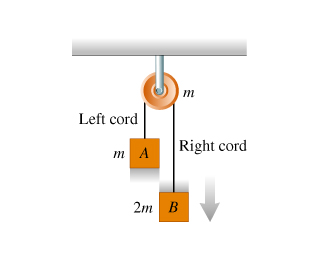# Problem: The figure below shows two blocks suspended by a cord over a pulley.(Figure 1)The mass of block B is twice the mass of block A, while the mass of the pulley is equal to the mass of block A. The blocks are let free to move and the cord moves on the pulley without slipping or stretching. There is no friction in the pulley axle, and the cord's weight can be ignored.What happens when block B moves downward?A) The Left cord pulls on the pulley with greater force than the right cordB) The left and right cord pull with equal force on the pulleyC) The right cord pulls on the pulley with greater force than the left cord

###### FREE Expert Solution

This problem requires us to explain how how torque affects the tension in a string.

Torque equations:

$\overline{){\mathbit{\Sigma }}{\mathbit{\tau }}{\mathbf{=}}{\mathbit{\alpha }}{\mathbit{I}}}$ α is the angular acceleration while I is the moment of inertia.

$\overline{){\mathbit{\tau }}{\mathbf{=}}{\mathbit{r}}{\mathbit{F}}}$

81% (124 ratings)###### Problem Details

The figure below shows two blocks suspended by a cord over a pulley.(Figure 1)The mass of block B is twice the mass of block A, while the mass of the pulley is equal to the mass of block A. The blocks are let free to move and the cord moves on the pulley without slipping or stretching. There is no friction in the pulley axle, and the cord's weight can be ignored.What happens when block B moves downward?

A) The Left cord pulls on the pulley with greater force than the right cord

B) The left and right cord pull with equal force on the pulley

C) The right cord pulls on the pulley with greater force than the left cord